# Number Sentence - Definition with Examples

The Complete K-5 Math Learning Program Built for Your Child

• 40 Million Kids

Loved by kids and parent worldwide

• 50,000 Schools

Trusted by teachers across schools

• Comprehensive Curriculum

Aligned to Common Core

Number sentence

A number sentence is a mathematical sentence, made up of numbers and signs.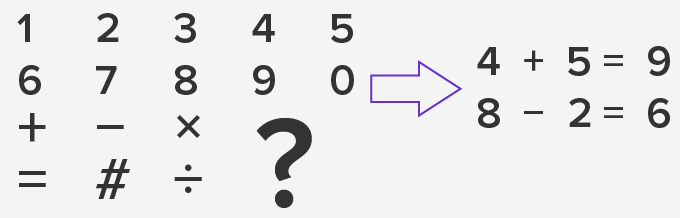The expressions given in  examples indicate equality or inequality.Types of Number Sentences

A number sentence can use any of the mathematical operations from addition, subtraction, multiplication to division. Symbols used in any number sentence vary depending upon what they indicate.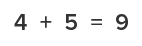Addition Sentence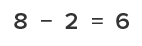Subtraction Sentence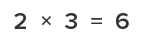Multiplication Sentence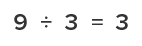Division Sentence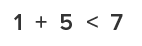Less than Sentence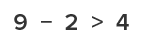Greater than Sentence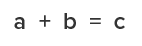Algebraic Sentence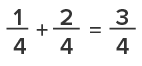Fraction Sentence

Number sentences can be true or they may not be true.

For example:

10 + 5 = 15. Here we are using the = sign which indicates a balance of both sides.

However, there could also be number sentences which say:

12 + 6 = 9 is not true, but 12 + 6 = 18 is true.

Therefore, a number sentence does not necessarily have to be true. However, every number sentence gives us information, and based on the information provided; it is possible to change the statement from false to true.

So, a number sentence contains numbers, mathematical operations, equal to or inequality sign and a number after the equality or inequality sign. If we remove any of these components, it will no longer be a number sentence.

For example:

10 + 8 > 15

However, if we write 10 + 8 it is not enough to understand what is the question to be solved.

If we write 10 + 8   15. It does not make any sense

If we write +   >, this does not make any sense too.

Number sentence problems can come in the form of word problems, asking the students to write the equation.

For example:  Mary has 10 strawberries. If Dan gives her 15 strawberries, how many strawberries does Mary have in total?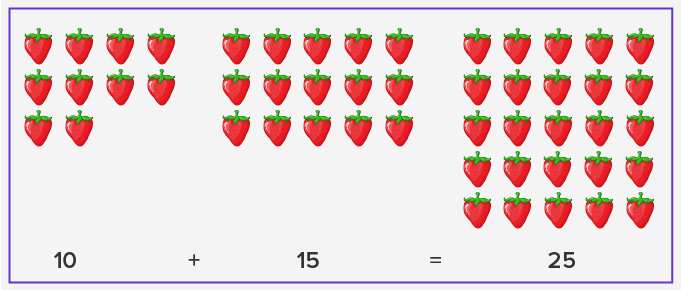Other examples of a problem for number sentence could be as follows:

20 +   ____   = 25

___   –  20 = 80

 Fun fact:  Number sentences are simply the numerical expression of a word problem.

Won Numerous Awards & Honors